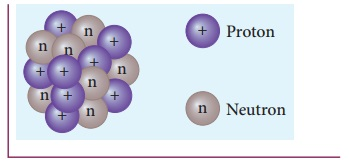Home | | Physics 12th Std | Size and density of the nucleus

# Size and density of the nucleus

The alpha particle scattering experiment and many other measurements using different methods have been carried out on the nuclei of various atoms.

Size and density of the nucleus

The alpha particle scattering experiment and many other measurements using different methods have been carried out on the nuclei of various atoms. The nuclei are found to be approximately spherical in shape. It is experimentally found that radius of nuclei for Z > 10, satisfies the following empirical formulaHere A is the mass number of the nucleus and the constant R0 = 1.2 F, where 1 F = 1 × 10–15 m. The unit fermi (F) is named after Enrico Fermi.

EXAMPLE 8.7

Calculate the radius of 19779Au nucleus.

Solution

According to the equation (8.19),

R = 1.2 × 10-15 × (197)1/3 = 6.97 × 10-15m

Or R = 6.97 F

EXAMPLE 8.8

Calculate the density of the nucleus with mass number A.

Solution

From equation (8.19), the radius of the nuclei satisfy the equation = R0A1/3. Then the volume of the nucleus

V = 4/3 πR3 = 4/3 πR3A

By ignoring the mass difference between the proton and neutron, the total mass of the nucleus having mass number A is equal to A.m where m is mass of the proton and is equal to 1.6726 x 10-27 kg.The above expression shows that the nuclear density is independent of the mass number A. In other words, all the nuclei (Z > 10) have the same density and it is an important characteristics of the nuclei.

We can calculate the numerical value of this density by substituting the corresponding values.It implies that nucleons are extremely tightly packed in the nucleus and compare this density with the density of water which is 103 kg m-3.

A single teaspoon of nuclear matter would weigh about trillion tons.

Tags : Nuclear Physics , 12th Physics : UNIT 9 : Atomic and Nuclear Physics
Study Material, Lecturing Notes, Assignment, Reference, Wiki description explanation, brief detail
12th Physics : UNIT 9 : Atomic and Nuclear Physics : Size and density of the nucleus | Nuclear Physics